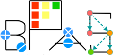Basic Polynomial Algebra Subprograms (BPAS)  v. 1.652
BPASTriangularSet< Field, RecursiveFieldPoly > Class Template Referenceabstract

An abstract class defining the interface of a triangular set. More...

`#include <polynomial.h>`

Simplified semantic inheritance diagram for BPASTriangularSet< Field, RecursiveFieldPoly >:Full inheritance diagram for BPASTriangularSet< Field, RecursiveFieldPoly >:
[legend]

## Public Member Functions

virtual BPASTriangularSet< Field, RecursiveFieldPoly > & operator= (const BPASTriangularSet< Field, RecursiveFieldPoly > &)=0

virtual BPASTriangularSet< Field, RecursiveFieldPoly > & operator= (BPASTriangularSet< Field, RecursiveFieldPoly > &&)=0

virtual int numberOfVariables () const =0

virtual std::vector< Symbolvariables () const =0

virtual RecursiveFieldPoly select (const Symbol &) const =0

virtual void lower (const Symbol &, BPASTriangularSet< Field, RecursiveFieldPoly > &) const =0

virtual void upper (const Symbol &, BPASTriangularSet< Field, RecursiveFieldPoly > &) const =0

virtual RecursiveFieldPoly pseudoDivide (const RecursiveFieldPoly &, std::vector< RecursiveFieldPoly > *, RecursiveFieldPoly *) const =0

virtual RecursiveFieldPoly normalForm (const RecursiveFieldPoly &, std::vector< RecursiveFieldPoly > *) const =0

## Detailed Description

### template<class Field, class RecursiveFieldPoly> class BPASTriangularSet< Field, RecursiveFieldPoly >

An abstract class defining the interface of a triangular set.

A BPASTriangularSet is templated by a BPASRecursivelyViewedPolynomial with coefficients in a BPASField.

The documentation for this class was generated from the following file: